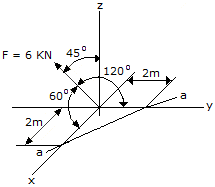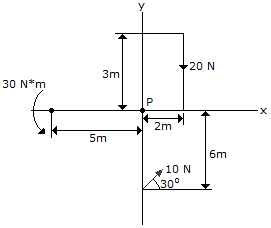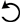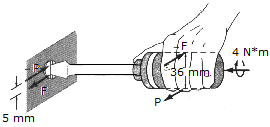# Engineering Mechanics - Force System Resultants

### Exercise :: Force System Resultants - General Questions

• Force System Resultants - General Questions
41.Determine the magnitude of the projection of the moment cause by the force about the aa axis.

 A. Maa = 16.97 kN-m B. Maa = 12.00 kN-m C. Maa = 6 kN-m D. Maa = 8.48 kN-m

Explanation:

No answer description available for this question. Let us discuss.

42.Replace the force and couple system by an equivalent single force and couple acting at point P.

 A. F = (-8.66i-15.00j) N, M = 50.0 N-mB. F = (8.66i-15.00j) N, M = 42.0 N-mC. F = (8.66i-15.00j) N, M = 50.0 N-mD. F = (-8.66i-15.00j) N, M = 42.0 N-mExplanation:

No answer description available for this question. Let us discuss.

43.A twist of 4 N-m is applied to the handle of the screwdriver. Resolve this couple moment into a pair of couple forces F exerted on the handle.

 A. F = 133 N B. F = 120 N C. F = 60 N D. F = 266 N